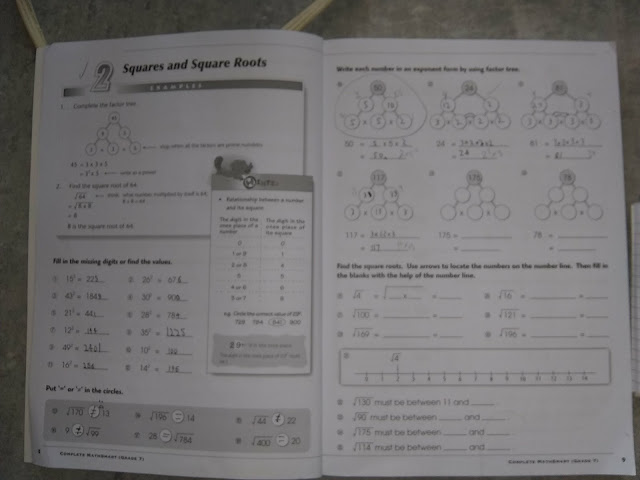## Wednesday, April 17, 2019

### Self-Directed Homeschooling

As part of our routine of homeschooling, on so-called "regular day"s, Kenny usually cracks open a lesson book at or above his contemporaries' grade level.  Yesterday he was exempted from any lessons as I had to do computer calls that took up the entire morning.  He comes along with me for most of these, and I feel that interacting with adults and watching me work count for an educational experience.

The day before this though was a regular day, and he worked away at his mathematics lesson on squares and square roots.  I was impressed at how many he tried to do in his head, but I did give him permission to use a calculator for ones that I didn't have memorized or couldn't do easily myself.This is what he's been working on lately.
We have a Google Home Mini, but for some reason, he prefers to use the calculator for things like this, as he also does for spelling.  Go figure.

Part way through the lesson, he noted that while 12^2 is 144, 21^2 is the correspondingly interesting value of 441.

He mused if other examples existed.  I quickly pointed out that 001^2 is 1, and 10^2 is 100.  I wonder just how impressive my mathematical feat was to him, as he was able to contain his admiration completely.

Grabbing a small notebook he used as a scratch pad, he began working his way through the squares from 1 to 99.  At least, this was his ambition.

I'm lazy efficient, and mused if it wouldn't be easier to write a computer programme to calculate these squares and simply print them out in a format that one could more quickly skim them.  It was in under an hour that he had written a python script that actually did the squares AS WELL AS the comparison.

I'll attach the script at the end, feel free to copy as you will.  Please note that while I'm a coder from old, I have no experience with Python - all of this coding was his from start to finish; I don't know how it works.

In case you're curious, it turns out that there are four of these relationships in a row, then three, followed by two and then a single outlier before the pattern vanishes.

Score one for homeschooling allowing him the ability to go down a side road that would rarely be permitted in a regular environment!

Here's his code:

s1 = 0
s2 = 0
s = 0
stbf = 0
while s < 99:
s = s1 + (s2 * 10)
st = s * s
if len(str(st)) == 1:
st1 = st
st2 = 0
st3 = 0
st4 = 0
elif len(str(st)) == 2:
st1 = str(st)
st2 = str(st)
st3 = 0
st4 = 0
elif len(str(st)) == 3:
st1 = str(st)
st2 = str(st)
st3 = str(st)
st4 = 0
elif len(str(st)) == 4:
st1 = str(st)
st2 = str(st)
st3 = str(st)
st4 = str(st)
sb = str(s1) + str(s2)
stb = int(sb) * int(sb)
if len(str(stb)) == 1:
stbf = "000" + str(stb)
elif len(str(stb)) == 2:
stbf = "00" + str(stb)
elif len(str(stb)) == 3:
stbf = "0" + str(stb)
elif len(str(stb)) == 4:
stbf = str(stb)

stbf1 = str(stbf)
stbf2 = str(stbf)
stbf3 = str(stbf)
stbf4 = str(stbf)
if len(str(stb)) == 1:
stbfb = int(stbf1)
elif len(str(stb)) == 2:
stbfb = int(stbf1 + stbf2)
elif len(str(stb)) == 3:
stbfb = int(stbf1 + stbf2 + stbf3)
elif len(str(stb)) == 4:
stbfb = int(stbf1 + stbf2 + stbf3 + stbf4)
a1 = int(str(st4) + str(st3) + str(st2) + str(st1))
a = "NO"
if int(a1) == int(stbfb):
a = "YES"
print str(s2) + str(s1) + "|" + str(st4) + str(st3) + str(st2) + str(st1) + "| |" + str(sb) + "|" + str(stbf) + "| |" + str(a)
s1 += 1
if s1 == 10:
s1 = 0
s2 += 1
while True:
a = a

#### 1 comment:

1.Does this code give you the same results? It's somewhat cleaner, but also might be making different assumptions than you were about what counts as a match. It's using python v2.7.

One feature of python is that you can index objects from either the start or the end. So if you have a list abc, you can use abc to return the first item or abc[-1] to return the last. Of course you can also get the last using abc[len(abc)-1].

Another thing you can do in python is convert a number to a string with a specific number of digits (for example this would print the string 0055): print '%04d' % 55

d1 = 0 # smaller digit
d2 = 0 # larger digit
v1 = 0 # value 1
v2 = 0 # value 2
while v1 < 100:
# squares
v1s = v1*v1
v2s = v2*v2

if len(str(v1s)) != len(str(v2s)):
match = 'NO'
else:
match = 'YES'
index = -1
for digit in str(v1s):
if str(v2s)[index] != digit:
match = 'NO'
break
index -= 1

# results
print '%02s : %02s : %04s : %04s : %s' % (v1, v2, v1s, v2s, match)

# increment
d1 += 1
if d1 >= 10:
d1 = 0
d2 += 1
v1 = d1 + 10*d2
v2 = d2 + 10*d1